# مصفوفة

المصفوفة Matrix هي دالة رياضية خطية تحول مجموعة بداية أي إنطلاق (مجال) إلى مجموعة وصول أو نهاية (مدى). مجموعة الإنطلاق و الوصول يمكن أن تكون متكونة من أعداد صحيحة أو عقدية أو أشعة من الأعداد كما يمكن أن تكون هاتين المجموعتان متكونة بدورها من دالات رياضية أو أشعة دالات رياضية. ويمكن أن نرمز للمصفوفة بمعقفين يكتب بينهما عناصر المصفوفة كما هو مبين أسفله:
${\begin{bmatrix}{a}_{11}&{a}_{12}&\cdots &{a}_{1n}\\{a}_{21}&{a}_{22}&\cdots &{a}_{2n}\\\vdots &\ddots &\ddots &\vdots \\{a}_{m1}&{a}_{m2}&\cdots &{a}_{mn}\end{bmatrix}}$حيث ${a}_{ij}$يمكن أن تكون أعدادا صحيحة أو مركبة كما يمكن أن تكون دالات رياضية.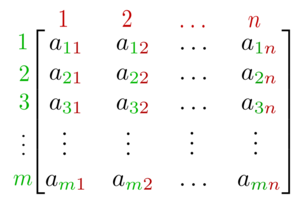An m × n matrix: the m rows are horizontal and the n columns are vertical. Each element of a matrix is often denoted by a variable with two subscripts. فعلى سبيل المثال، a2,1 represents the element at the second row and first column of the matrix.

نظرية المصفوفات هي فرع الرياضيات الذي يركز على دراسة المصفوفات. فعليا يعتبر أحد فروع الجبر الخطي, ثم نمى ليغطي موضوعات ذات علاقة بنظرية المخططات والجبر, والتوافقيات والإحصاء.

. . . . . . . . . . . . . . . . . . . . . . . . . . . . . . . . . . . . . . . . . . . . . . . . . . . . . . . . . . . . . . . . . . . . . . . . . . . . . . . . . . . . . . . . . . . . . . . . . . . . . . . . . . . . . . . . . . . . . . . . . . . . . . . . . . . . . . . . . . . . . . . . . . . . . . . . . . . . . . . . . . . . . . . .

## مثال على تحويل من مجموعة إنطلاق إلى مجموعة وصول

لنعتبر مثلا الشعاع التالي:
$V={\begin{bmatrix}{s}_{1}\\{s}_{2}\\{s}_{3}\\{s}_{4}\end{bmatrix}}\in {R}^{4}$
و المصفوفة التالية: $A={\begin{bmatrix}{a}_{11}&{a}_{12}&{a}_{13}&{a}_{14}\\{a}_{21}&{a}_{22}&{a}_{23}&{a}_{24}\end{bmatrix}}$

عملية تحويل الشعاع تتم على النحو التالي:
$X=A*V={\begin{bmatrix}{a}_{11}&{a}_{12}&{a}_{13}&{a}_{14}\\{a}_{21}&{a}_{22}&{a}_{23}&{a}_{24}\end{bmatrix}}{\begin{bmatrix}{s}_{1}\\{s}_{2}\\{s}_{3}\\{s}_{4}\end{bmatrix}}={\begin{bmatrix}{a}_{11}{s}_{1}+{a}_{12}{s}_{2}+{a}_{13}{s}_{3}+{a}_{14}{a}_{14}\\{a}_{21}{s}_{1}+{a}_{22}{s}_{2}+{a}_{23}{s}_{3}+{a}_{24}{s}_{4}\end{bmatrix}}$

وهكذا نكون قد حولنا شعاعا V ينتمي إلى ${R}^{4}$  إلى شعاع X ينتمي إلى ال ${R}^{2}$ . أما عامة إذا كانت المصفوفة تحتوي على عدد m من الأسطر و n من الأعمدة فإنها تحول مجموعة الإنطلاق المكونة من أشعة تنتمي إلى ال ${K}^{n}$  إلى مجموعة الوصول المتكونة من أشعة تنتمي إلى ال ${K}^{m}$ .
كما يمكن إعتبار المصفوفات نوعا خاصا من التنسورات ألا وهي التنسورات من الدرجة الثانية

## العمليات الأساسية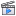How to organize, add and multiply matrices - Bill Shillito, TED ED

There are a number of basic operations that can be applied to modify matrices, called matrix addition, scalar multiplication, transposition, matrix multiplication, row operations, and submatrix.

### Addition, scalar multiplication, and transposition

Operation Definition Example
Addition The sum A+B of two m-by-n matrices A and B is calculated entrywise:
(A + B)i,j = Ai,j + Bi,j, where 1 ≤ im and 1 ≤ jn.

${\begin{bmatrix}1&3&1\\1&0&0\end{bmatrix}}+{\begin{bmatrix}0&0&5\\7&5&0\end{bmatrix}}={\begin{bmatrix}1+0&3+0&1+5\\1+7&0+5&0+0\end{bmatrix}}={\begin{bmatrix}1&3&6\\8&5&0\end{bmatrix}}$

Scalar multiplication The product cA of a number c (also called a scalar in the parlance of abstract algebra) and a matrix A is computed by multiplying every entry of A by c:
(cA)i,j = c · Ai,j.

This operation is called scalar multiplication, but its result is not named "scalar product" to avoid confusion, since "scalar product" is sometimes used as a synonym for "inner product".

$2\cdot {\begin{bmatrix}1&8&-3\\4&-2&5\end{bmatrix}}={\begin{bmatrix}2\cdot 1&2\cdot 8&2\cdot -3\\2\cdot 4&2\cdot -2&2\cdot 5\end{bmatrix}}={\begin{bmatrix}2&16&-6\\8&-4&10\end{bmatrix}}$
Transposition The transpose of an m-by-n matrix A is the n-by-m matrix AT (also denoted Atr or tA) formed by turning rows into columns and vice versa:
(AT)i,j = Aj,i.
${\begin{bmatrix}1&2&3\\0&-6&7\end{bmatrix}}^{\mathrm {T} }={\begin{bmatrix}1&0\\2&-6\\3&7\end{bmatrix}}$

Familiar properties of numbers extend to these operations of matrices: for example, addition is commutative, that is, the matrix sum does not depend on the order of the summands: A + B = B + A. The transpose is compatible with addition and scalar multiplication, as expressed by (cA)T = c(AT) and (A + B)T = AT + BT. Finally, (AT)T = A.

### ضرب المصفوفات

يتم ضرب مصفوفة في أخرى ولكن بتواجد الشرط الآتي : أن يكون عدد الأعمدة بالمصفوفة الأولى يساوي عدد الصفوف بالمصفوفة الثانية. يتم ضرب المصفوفات كالتالي : الصف الأول بالعمود الأول ثم الصف الثاني بالعمود الثاني .......إلخ،وينتج من ضرب الصف الأول بالعمود الأول العدد الأول بالمصفوفة الناتجة. ونضرب العدد الأول بالصف بالعدد الأول بالعمود.

Multiplication of two matrices is defined if and only if the number of columns of the left matrix is the same as the number of rows of the right matrix. If A is an m-by-n matrix and B is an n-by-p matrix, then their matrix product AB is the m-by-p matrix whose entries are given by dot product of the corresponding row of A and the corresponding column of B:

$[\mathbf {AB} ]_{i,j}=a_{i,1}b_{1,j}+a_{i,2}b_{2,j}+\cdots +a_{i,n}b_{n,j}=\sum _{r=1}^{n}a_{i,r}b_{r,j},$

where 1 ≤ im and 1 ≤ jp. For example, the underlined entry 2340 in the product is calculated as (2 × 1000) + (3 × 100) + (4 × 10) = 2340:

{\begin{aligned}{\begin{bmatrix}{\underline {2}}&{\underline {3}}&{\underline {4}}\\1&0&0\\\end{bmatrix}}{\begin{bmatrix}0&{\underline {1000}}\\1&{\underline {100}}\\0&{\underline {10}}\\\end{bmatrix}}&={\begin{bmatrix}3&{\underline {2340}}\\0&1000\\\end{bmatrix}}.\end{aligned}}

Matrix multiplication satisfies the rules (AB)C = A(BC) (associativity), and (A + B)C = AC + BC as well as C(A + B) = CA + CB (left and right distributivity), whenever the size of the matrices is such that the various products are defined. The product AB may be defined without BA being defined, namely if A and B are m-by-n and n-by-k matrices, respectively, and mk. Even if both products are defined, they need not be equal, that is, generally

ABBA,

that is, matrix multiplication is not commutative, in marked contrast to (rational, real, or complex) numbers whose product is independent of the order of the factors. An example of two matrices not commuting with each other is:

${\begin{bmatrix}1&2\\3&4\\\end{bmatrix}}{\begin{bmatrix}0&1\\0&0\\\end{bmatrix}}={\begin{bmatrix}0&1\\0&3\\\end{bmatrix}},$

whereas

${\begin{bmatrix}0&1\\0&0\\\end{bmatrix}}{\begin{bmatrix}1&2\\3&4\\\end{bmatrix}}={\begin{bmatrix}3&4\\0&0\\\end{bmatrix}}.$

Besides the ordinary matrix multiplication just described, there exist other less frequently used operations on matrices that can be considered forms of multiplication, such as the Hadamard product and the Kronecker product. They arise in solving matrix equations such as the Sylvester equation.

### Row operations

There are three types of row operations:

2. row multiplication, that is multiplying all entries of a row by a non-zero constant;
3. row switching, that is interchanging two rows of a matrix;

These operations are used in a number of ways, including solving linear equations and finding matrix inverses.

### Submatrix

A submatrix of a matrix is obtained by deleting any collection of rows and/or columns. For example, from the following 3-by-4 matrix, we can construct a 2-by-3 submatrix by removing row 3 and column 2:

$\mathbf {A} ={\begin{bmatrix}1&\color {red}{2}&3&4\\5&\color {red}{6}&7&8\\\color {red}{9}&\color {red}{10}&\color {red}{11}&\color {red}{12}\end{bmatrix}}\rightarrow {\begin{bmatrix}1&3&4\\5&7&8\end{bmatrix}}.$

The minors and cofactors of a matrix are found by computing the determinant of certain submatrices.

A principal submatrix is a square submatrix obtained by removing certain rows and columns. The definition varies from author to author. According to some authors, a principal submatrix is a submatrix in which the set of row indices that remain is the same as the set of column indices that remain. Other authors define a principal submatrix as one in which the first k rows and columns, for some number k, are the ones that remain; this type of submatrix has also been called a leading principal submatrix.

## المعادلات الخطية

Matrices can be used to compactly write and work with multiple linear equations, that is, systems of linear equations. For example, if A is an m-by-n matrix, x designates a column vector (that is, n×1-matrix) of n variables x1, x2, ..., xn, and b is an m×1-column vector, then the matrix equation

$\mathbf {Ax} =\mathbf {b}$

is equivalent to the system of linear equations

{\begin{aligned}a_{1,1}x_{1}+a_{1,2}x_{2}+&\cdots +a_{1,n}x_{n}=b_{1}\\&\ \ \vdots \\a_{m,1}x_{1}+a_{m,2}x_{2}+&\cdots +a_{m,n}x_{n}=b_{m}\end{aligned}}

Using matrices, this can be solved more compactly than would be possible by writing out all the equations separately. If n = m and the equations are independent, this can be done by writing

$\mathbf {x} =\mathbf {A} ^{-1}\mathbf {b}$

where A−1 is the inverse matrix of A. If A has no inverse, solutions if any can be found using its generalized inverse.

## التحولات الخطية

The vectors represented by a 2-by-2 matrix correspond to the sides of a unit square transformed into a parallelogram.

Matrices and matrix multiplication reveal their essential features when related to linear transformations, also known as linear maps. A real m-by-n matrix A gives rise to a linear transformation RnRm mapping each vector x in Rn to the (matrix) product Ax, which is a vector in Rm. Conversely, each linear transformation f: RnRm arises from a unique m-by-n matrix A: explicitly, the (i, j)-entry of A is the ith coordinate of f(ej), where ej = (0,...,0,1,0,...,0) is the unit vector with 1 in the jth position and 0 elsewhere. The matrix A is said to represent the linear map f, and A is called the transformation matrix of f.

For example, the 2×2 matrix

$\mathbf {A} ={\begin{bmatrix}a&c\\b&d\end{bmatrix}}$

can be viewed as the transform of the unit square into a parallelogram with vertices at (0, 0), (a, b), (a + c, b + d), and (c, d). The parallelogram pictured at the right is obtained by multiplying A with each of the column vectors ${\begin{bmatrix}0\\0\end{bmatrix}},{\begin{bmatrix}1\\0\end{bmatrix}},{\begin{bmatrix}1\\1\end{bmatrix}}$ , and ${\begin{bmatrix}0\\1\end{bmatrix}}$  in turn. These vectors define the vertices of the unit square.

The following table shows a number of 2-by-2 matrices with the associated linear maps of R2. The blue original is mapped to the green grid and shapes. The origin (0,0) is marked with a black point.

 Horizontal shear with m = 1.25. Reflection through the vertical axis Squeeze mapping with r = 3/2 Scaling by a factor of 3/2 Rotation by π/6 = 30° ${\begin{bmatrix}1&1.25\\0&1\end{bmatrix}}$${\begin{bmatrix}-1&0\\0&1\end{bmatrix}}$${\begin{bmatrix}{\frac {3}{2}}&0\\0&{\frac {2}{3}}\end{bmatrix}}$${\begin{bmatrix}{\frac {3}{2}}&0\\0&{\frac {3}{2}}\end{bmatrix}}$${\begin{bmatrix}\cos \left({\frac {\pi }{6}}\right)&-\sin \left({\frac {\pi }{6}}\right)\\\sin \left({\frac {\pi }{6}}\right)&\cos \left({\frac {\pi }{6}}\right)\end{bmatrix}}$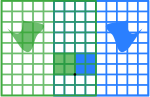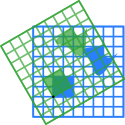Under the 1-to-1 correspondence between matrices and linear maps, matrix multiplication corresponds to composition of maps: if a k-by-m matrix B represents another linear map g: RmRk, then the composition gf is represented by BA since

(gf)(x) = g(f(x)) = g(Ax) = B(Ax) = (BA)x.

The last equality follows from the above-mentioned associativity of matrix multiplication.

The rank of a matrix A is the maximum number of linearly independent row vectors of the matrix, which is the same as the maximum number of linearly independent column vectors. Equivalently it is the dimension of the image of the linear map represented by A. The rank–nullity theorem states that the dimension of the kernel of a matrix plus the rank equals the number of columns of the matrix.

. . . . . . . . . . . . . . . . . . . . . . . . . . . . . . . . . . . . . . . . . . . . . . . . . . . . . . . . . . . . . . . . . . . . . . . . . . . . . . . . . . . . . . . . . . . . . . . . . . . . . . . . . . . . . . . . . . . . . . . . . . . . . . . . . . . . . . . . . . . . . . . . . . . . . . . . . . . . . . . . . . . . . . . .

## المصفوفة المربعة

A square matrix is a matrix with the same number of rows and columns. An n-by-n matrix is known as a square matrix of order n. Any two square matrices of the same order can be added and multiplied. The entries aii form the main diagonal of a square matrix. They lie on the imaginary line that runs from the top left corner to the bottom right corner of the matrix.

### الأنواع الرئيسية

الاسم أمثلة فيها n = 3
Diagonal matrix ${\begin{bmatrix}a_{11}&0&0\\0&a_{22}&0\\0&0&a_{33}\\\end{bmatrix}}$
Lower triangular matrix ${\begin{bmatrix}a_{11}&0&0\\a_{21}&a_{22}&0\\a_{31}&a_{32}&a_{33}\\\end{bmatrix}}$
Upper triangular matrix ${\begin{bmatrix}a_{11}&a_{12}&a_{13}\\0&a_{22}&a_{23}\\0&0&a_{33}\\\end{bmatrix}}$

#### المصفوفة القطرية والمثلثة

If all entries of A below the main diagonal are zero, A is called an upper triangular matrix. Similarly if all entries of A above the main diagonal are zero, A is called a lower triangular matrix. If all entries outside the main diagonal are zero, A is called a diagonal matrix.

#### Identity matrix

The identity matrix In of size n is the n-by-n matrix in which all the elements on the main diagonal are equal to 1 and all other elements are equal to 0, for example,

$\mathbf {I} _{1}={\begin{bmatrix}1\end{bmatrix}},\ \mathbf {I} _{2}={\begin{bmatrix}1&0\\0&1\end{bmatrix}},\ \cdots ,\ \mathbf {I} _{n}={\begin{bmatrix}1&0&\cdots &0\\0&1&\cdots &0\\\vdots &\vdots &\ddots &\vdots \\0&0&\cdots &1\end{bmatrix}}$

It is a square matrix of order n, and also a special kind of diagonal matrix. It is called an identity matrix because multiplication with it leaves a matrix unchanged:

AIn = ImA = A for any m-by-n matrix A.

A nonzero scalar multiple of an identity matrix is called a scalar matrix. If the matrix entries come from a field, the scalar matrices form a group, under matrix multiplication, that is isomorphic to the multiplicative group of nonzero elements of the field.

#### Symmetric or skew-symmetric matrix

A square matrix A that is equal to its transpose, that is, A = AT, is a symmetric matrix. If instead, A is equal to the negative of its transpose, that is, A = −AT, then A is a skew-symmetric matrix. In complex matrices, symmetry is often replaced by the concept of Hermitian matrices, which satisfy A = A, where the star or asterisk denotes the conjugate transpose of the matrix, that is, the transpose of the complex conjugate of A.

By the spectral theorem, real symmetric matrices and complex Hermitian matrices have an eigenbasis; that is, every vector is expressible as a linear combination of eigenvectors. In both cases, all eigenvalues are real. This theorem can be generalized to infinite-dimensional situations related to matrices with infinitely many rows and columns, see below.

#### Invertible matrix and its inverse

A square matrix A is called invertible or non-singular if there exists a matrix B such that

AB = BA = In ,

where In is the n×n identity matrix with 1s on the main diagonal and 0s elsewhere. If B exists, it is unique and is called the inverse matrix of A, denoted A−1.

#### Definite matrix

Positive definite matrix Indefinite matrix
${\begin{bmatrix}{\frac {1}{4}}&0\\0&1\\\end{bmatrix}}$  ${\begin{bmatrix}{\frac {1}{4}}&0\\0&-{\frac {1}{4}}\end{bmatrix}}$
Q(x, y) = 1/4 x2 + y2 Q(x, y) = 1/4 x2 − 1/4 y2

Points such that Q(x,y)=1
(Ellipse).

Points such that Q(x,y)=1
(Hyperbola).

A symmetric n×n-matrix A is called positive-definite if the associated quadratic form

fString Module Error: function rep expects a number as second parameter, received "hair"(x) = xTA x

has a positive value for every nonzero vector x in Rn. If fString Module Error: function rep expects a number as second parameter, received "hair"(x) only yields negative values then A is negative-definite; if f does produce both negative and positive values then A is indefinite. If the quadratic form f yields only non-negative values (positive or zero), the symmetric matrix is called positive-semidefinite (or if only non-positive values, then negative-semidefinite); hence the matrix is indefinite precisely when it is neither positive-semidefinite nor negative-semidefinite.

A symmetric matrix is positive-definite if and only if all its eigenvalues are positive, that is, the matrix is positive-semidefinite and it is invertible. The table at the right shows two possibilities for 2-by-2 matrices.

Allowing as input two different vectors instead yields the bilinear form associated to A:

BA (x, y) = xTAy.

#### المصفوفة العمودية Orthogonal

An orthogonal matrix is a square matrix with real entries whose columns and rows are orthogonal unit vectors (that is, orthonormal vectors). Equivalently, a matrix A is orthogonal if its transpose is equal to its inverse:

$\mathbf {A} ^{\mathrm {T} }=\mathbf {A} ^{-1},\,$

which entails

$\mathbf {A} ^{\mathrm {T} }\mathbf {A} =\mathbf {A} \mathbf {A} ^{\mathrm {T} }=\mathbf {I} _{n},$

where In is the identity matrix of size n.

An orthogonal matrix A is necessarily invertible (with inverse A−1 = AT), unitary (A−1 = A*), and normal (A*A = AA*). The determinant of any orthogonal matrix is either +1 or −1. A special orthogonal matrix is an orthogonal matrix with determinant +1. As a linear transformation, every orthogonal matrix with determinant +1 is a pure rotation without reflection, i.e., the transformation preserves the orientation of the transformed structure, while every orthogonal matrix with determinant -1 reverses the orientation, i.e., is a composition of a pure reflection and a (possibly null) rotation. The identity matrices have determinant 1, and are pure rotations by an angle zero.

The complex analogue of an orthogonal matrix is a unitary matrix.

### العمليات الرئيسية

. . . . . . . . . . . . . . . . . . . . . . . . . . . . . . . . . . . . . . . . . . . . . . . . . . . . . . . . . . . . . . . . . . . . . . . . . . . . . . . . . . . . . . . . . . . . . . . . . . . . . . . . . . . . . . . . . . . . . . . . . . . . . . . . . . . . . . . . . . . . . . . . . . . . . . . . . . . . . . . . . . . . . . . .

#### Trace

The trace, tr(A) of a square matrix A is the sum of its diagonal entries. While matrix multiplication is not commutative as mentioned above, the trace of the product of two matrices is independent of the order of the factors:

tr(AB) = tr(BA).

This is immediate from the definition of matrix multiplication:

$\operatorname {tr} (\mathbf {AB} )=\sum _{i=1}^{m}\sum _{j=1}^{n}a_{ij}b_{ji}=\operatorname {tr} (\mathbf {BA} ).$

It follows that the trace of the product of more than two matrices is independent of cyclic permutations of the matrices, however this does not in general apply for arbitrary permutations (for example, tr(ABC) ≠ tr(BAC), in general). Also, the trace of a matrix is equal to that of its transpose, that is,

tr(A) = tr(AT).

#### المحددة

A linear transformation on R2 given by the indicated matrix. The determinant of this matrix is −1, as the area of the green parallelogram at the right is 1, but the map reverses the orientation, since it turns the counterclockwise orientation of the vectors to a clockwise one.

The determinant det(A) or |A| of a square matrix A is a number encoding certain properties of the matrix. A matrix is invertible if and only if its determinant is nonzero. Its absolute value equals the area (in R2) or volume (in R3) of the image of the unit square (or cube), while its sign corresponds to the orientation of the corresponding linear map: the determinant is positive if and only if the orientation is preserved.

The determinant of 2-by-2 matrices is given by

$\det {\begin{bmatrix}a&b\\c&d\end{bmatrix}}=ad-bc.$

The determinant of 3-by-3 matrices involves 6 terms (rule of Sarrus). The more lengthy Leibniz formula generalises these two formulae to all dimensions.

The determinant of a product of square matrices equals the product of their determinants:

det(AB) = det(A) · det(B).

Adding a multiple of any row to another row, or a multiple of any column to another column, does not change the determinant. Interchanging two rows or two columns affects the determinant by multiplying it by −1. Using these operations, any matrix can be transformed to a lower (or upper) triangular matrix, and for such matrices the determinant equals the product of the entries on the main diagonal; this provides a method to calculate the determinant of any matrix. Finally, the Laplace expansion expresses the determinant in terms of minors, that is, determinants of smaller matrices. This expansion can be used for a recursive definition of determinants (taking as starting case the determinant of a 1-by-1 matrix, which is its unique entry, or even the determinant of a 0-by-0 matrix, which is 1), that can be seen to be equivalent to the Leibniz formula. Determinants can be used to solve linear systems using Cramer's rule, where the division of the determinants of two related square matrices equates to the value of each of the system's variables.

#### Eigenvalues and eigenvectors

A number λ and a non-zero vector v satisfying

Av = λv

are called an eigenvalue and an eigenvector of A, respectively. The number λ is an eigenvalue of an n×n-matrix A if and only if A−λIn is not invertible, which is equivalent to

$\det({\mathsf {A}}-\lambda {\mathsf {I}})=0.$ 

The polynomial pA in an indeterminate X given by evaluation the determinant det(XInA) is called the characteristic polynomial of A. It is a monic polynomial of degree n. Therefore the polynomial equation pA(λ) = 0 has at most n different solutions, that is, eigenvalues of the matrix. They may be complex even if the entries of A are real. According to the Cayley–Hamilton theorem, pA(A) = 0, that is, the result of substituting the matrix itself into its own characteristic polynomial yields the zero matrix.

## الهامش

1. ^ "How to organize, add and multiply matrices - Bill Shillito". TED ED. Retrieved April 6, 2013.
2. ^ Brown 1991, Definition I.2.1 (addition), Definition I.2.4 (scalar multiplication), and Definition I.2.33 (transpose)
3. ^ Brown 1991, Theorem I.2.6
4. ^ Brown 1991, Definition I.2.20
5. ^ Brown 1991, Theorem I.2.24
6. ^ Horn & Johnson 1985, Ch. 4 and 5
7. ^ Bronson (1970, p. 16)
8. ^ Kreyszig (1972, p. 220)
9. ^ أ ب Protter & Morrey (1970, p. 869)
10. ^ Kreyszig (1972, pp. 241,244)
11. ^ Schneider, Hans; Barker, George Phillip (2012), Matrices and Linear Algebra, Dover Books on Mathematics, Courier Dover Corporation, p. 251, ISBN 9780486139302 .
12. ^ Perlis, Sam (1991), Theory of Matrices, Dover books on advanced mathematics, Courier Dover Corporation, p. 103, ISBN 9780486668109 .
13. ^ Anton, Howard (2010), Elementary Linear Algebra (10th ed.), John Wiley & Sons, p. 414, ISBN 9780470458211 .
14. ^ Horn, Roger A.; Johnson, Charles R. (2012), Matrix Analysis (2nd ed.), Cambridge University Press, p. 17, ISBN 9780521839402 .
15. ^ Brown 1991, I.2.21 and 22
16. ^ Greub 1975, Section III.2
17. ^ Brown 1991, Definition II.3.3
18. ^ Greub 1975, Section III.1
19. ^ Brown 1991, Theorem II.3.22
20. ^ Horn & Johnson 1985, Theorem 2.5.6
21. ^ Brown 1991, Definition I.2.28
22. ^ Brown 1991, Definition I.5.13
23. ^ Horn & Johnson 1985, Chapter 7
24. ^ Horn & Johnson 1985, Theorem 7.2.1
25. ^ Horn & Johnson 1985, Example 4.0.6, p. 169
26. ^ Brown 1991, Definition III.2.1
27. ^ Brown 1991, Theorem III.2.12
28. ^ Brown 1991, Corollary III.2.16
29. ^ Mirsky 1990, Theorem 1.4.1
30. ^ Brown 1991, Theorem III.3.18
31. ^ Eigen means "own" in German and in Dutch.
32. ^ Brown 1991, Definition III.4.1
33. ^ Brown 1991, Definition III.4.9
34. ^ Brown 1991, Corollary III.4.10

## المراجع

• Anton, Howard (1987), Elementary Linear Algebra (5th ed.), New York: Wiley, ISBN 0-471-84819-0
• Arnold, Vladimir I.; Cooke, Roger (1992), Ordinary differential equations, Berlin, DE; New York, NY: Springer-Verlag, ISBN 978-3-540-54813-3
• Artin, Michael (1991), Algebra, Prentice Hall, ISBN 978-0-89871-510-1
• Association for Computing Machinery (1979), Computer Graphics, Tata McGraw–Hill, ISBN 978-0-07-059376-3
• Baker, Andrew J. (2003), Matrix Groups: An Introduction to Lie Group Theory, Berlin, DE; New York, NY: Springer-Verlag, ISBN 978-1-85233-470-3
• Bau III, David; Trefethen, Lloyd N. (1997), Numerical linear algebra, Philadelphia, PA: Society for Industrial and Applied Mathematics, ISBN 978-0-89871-361-9
• Beauregard, Raymond A.; Fraleigh, John B. (1973), A First Course In Linear Algebra: with Optional Introduction to Groups, Rings, and Fields, Boston: Houghton Mifflin Co., ISBN 0-395-14017-X
• Bretscher, Otto (2005), Linear Algebra with Applications (3rd ed.), Prentice Hall
• Bronson, Richard (1970), Matrix Methods: An Introduction, New York: Academic Press
• Bronson, Richard (1989), Schaum's outline of theory and problems of matrix operations, New York: McGraw–Hill, ISBN 978-0-07-007978-6
• Brown, William C. (1991), Matrices and vector spaces, New York, NY: Marcel Dekker, ISBN 978-0-8247-8419-5
• Coburn, Nathaniel (1955), Vector and tensor analysis, New York, NY: Macmillan, OCLC 1029828
• Conrey, J. Brian (2007), Ranks of elliptic curves and random matrix theory, Cambridge University Press, ISBN 978-0-521-69964-8
• Fraleigh, John B. (1976), A First Course In Abstract Algebra (2nd ed.), Reading: Addison-Wesley, ISBN 0-201-01984-1
• Fudenberg, Drew; Tirole, Jean (1983), Game Theory, MIT Press
• Gilbarg, David; Trudinger, Neil S. (2001), Elliptic partial differential equations of second order (2nd ed.), Berlin, DE; New York, NY: Springer-Verlag, ISBN 978-3-540-41160-4
• Godsil, Chris; Royle, Gordon (2004), Algebraic Graph Theory, Graduate Texts in Mathematics, 207, Berlin, DE; New York, NY: Springer-Verlag, ISBN 978-0-387-95220-8
• Golub, Gene H.; Van Loan, Charles F. (1996), Matrix Computations (3rd ed.), Johns Hopkins, ISBN 978-0-8018-5414-9
• Greub, Werner Hildbert (1975), Linear algebra, Graduate Texts in Mathematics, Berlin, DE; New York, NY: Springer-Verlag, ISBN 978-0-387-90110-7
• Halmos, Paul Richard (1982), A Hilbert space problem book, Graduate Texts in Mathematics, 19 (2nd ed.), Berlin, DE; New York, NY: Springer-Verlag, ISBN 978-0-387-90685-0
• Horn, Roger A.; Johnson, Charles R. (1985), Matrix Analysis, Cambridge University Press, ISBN 978-0-521-38632-6
• Householder, Alston S. (1975), The theory of matrices in numerical analysis, New York, NY: Dover Publications
• Kreyszig, Erwin (1972), Advanced Engineering Mathematics (3rd ed.), New York: Wiley, ISBN 0-471-50728-8 .
• Krzanowski, Wojtek J. (1988), Principles of multivariate analysis, Oxford Statistical Science Series, 3, The Clarendon Press Oxford University Press, ISBN 978-0-19-852211-9
• Itô, Kiyosi, ed. (1987), Encyclopedic dictionary of mathematics. Vol. I-IV (2nd ed.), MIT Press, ISBN 978-0-262-09026-1
• Lang, Serge (1969), Analysis II, Addison-Wesley
• Lang, Serge (1987a), Calculus of several variables (3rd ed.), Berlin, DE; New York, NY: Springer-Verlag, ISBN 978-0-387-96405-8
• Lang, Serge (1987b), Linear algebra, Berlin, DE; New York, NY: Springer-Verlag, ISBN 978-0-387-96412-6
• قالب:Lang Algebra
• Latouche, Guy; Ramaswami, Vaidyanathan (1999), Introduction to matrix analytic methods in stochastic modeling (1st ed.), Philadelphia, PA: Society for Industrial and Applied Mathematics, ISBN 978-0-89871-425-8
• Manning, Christopher D.; Schütze, Hinrich (1999), Foundations of statistical natural language processing, MIT Press, ISBN 978-0-262-13360-9
• Mehata, K. M.; Srinivasan, S. K. (1978), Stochastic processes, New York, NY: McGraw–Hill, ISBN 978-0-07-096612-3
• Mirsky, Leonid (1990), An Introduction to Linear Algebra, Courier Dover Publications, ISBN 978-0-486-66434-7
• Nering, Evar D. (1970), Linear Algebra and Matrix Theory (2nd ed.), New York: Wiley
• Nocedal, Jorge; Wright, Stephen J. (2006), Numerical Optimization (2nd ed.), Berlin, DE; New York, NY: Springer-Verlag, p. 449, ISBN 978-0-387-30303-1
• Oualline, Steve (2003), Practical C++ programming, O'Reilly, ISBN 978-0-596-00419-4
• Press, William H.; Flannery, Brian P.; Teukolsky, Saul A.; Vetterling, William T. (1992), "LU Decomposition and Its Applications", Numerical Recipes in FORTRAN: The Art of Scientific Computing (2nd ed.), Cambridge University Press, pp. 34–42, Archived from the original on 2009-09-06
• Protter, Murray H.; Morrey, Jr., Charles B. (1970), College Calculus with Analytic Geometry (2nd ed.), Reading: Addison-Wesley
• Punnen, Abraham P.; Gutin, Gregory (2002), The traveling salesman problem and its variations, Boston, MA: Kluwer Academic Publishers, ISBN 978-1-4020-0664-7
• Reichl, Linda E. (2004), The transition to chaos: conservative classical systems and quantum manifestations, Berlin, DE; New York, NY: Springer-Verlag, ISBN 978-0-387-98788-0
• Rowen, Louis Halle (2008), Graduate Algebra: noncommutative view, Providence, RI: American Mathematical Society, ISBN 978-0-8218-4153-2
• Šolin, Pavel (2005), Partial Differential Equations and the Finite Element Method, Wiley-Interscience, ISBN 978-0-471-76409-0
• Stinson, Douglas R. (2005), Cryptography, Discrete Mathematics and its Applications, Chapman & Hall/CRC, ISBN 978-1-58488-508-5
• Stoer, Josef; Bulirsch, Roland (2002), Introduction to Numerical Analysis (3rd ed.), Berlin, DE; New York, NY: Springer-Verlag, ISBN 978-0-387-95452-3
• Ward, J. P. (1997), Quaternions and Cayley numbers, Mathematics and its Applications, 403, Dordrecht, NL: Kluwer Academic Publishers Group, doi:10.1007/978-94-011-5768-1, ISBN 978-0-7923-4513-8
• Wolfram, Stephen (2003), The Mathematica Book (5th ed.), Champaign, IL: Wolfram Media, ISBN 978-1-57955-022-6

### مراجع فيزياء

• Bohm, Arno (2001), Quantum Mechanics: Foundations and Applications, Springer, ISBN 0-387-95330-2
• Burgess, Cliff; Moore, Guy (2007), The Standard Model. A Primer, Cambridge University Press, ISBN 0-521-86036-9
• Guenther, Robert D. (1990), Modern Optics, John Wiley, ISBN 0-471-60538-7
• Itzykson, Claude; Zuber, Jean-Bernard (1980), Quantum Field Theory, McGraw–Hill, ISBN 0-07-032071-3
• Riley, Kenneth F.; Hobson, Michael P.; Bence, Stephen J. (1997), Mathematical methods for physics and engineering, Cambridge University Press, ISBN 0-521-55506-X
• Schiff, Leonard I. (1968), Quantum Mechanics (3rd ed.), McGraw–Hill
• Weinberg, Steven (1995), The Quantum Theory of Fields. Volume I: Foundations, Cambridge University Press, ISBN 0-521-55001-7
• Wherrett, Brian S. (1987), Group Theory for Atoms, Molecules and Solids, Prentice–Hall International, ISBN 0-13-365461-3
• Zabrodin, Anton; Brezin, Édouard; Kazakov, Vladimir; Serban, Didina; Wiegmann, Paul (2006), Applications of Random Matrices in Physics (NATO Science Series II: Mathematics, Physics and Chemistry), Berlin, DE; New York, NY: Springer-Verlag, ISBN 978-1-4020-4530-1

## وصلات خارجية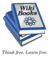هناك كتاب ، جبر خطي، في معرفة الكتب.

Encyclopedic articles
History
Online books
Online matrix calculators